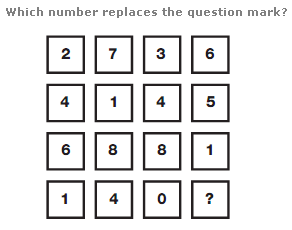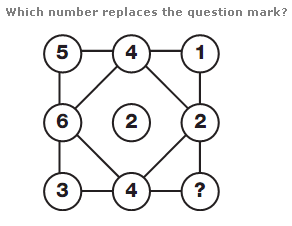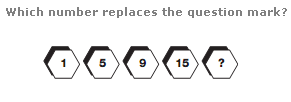# Puzzles - Number puzzles

Exercise : Number puzzles
64.:
9
Explanation
:
Reading each horizontal line as a 4 digit number, add together the top two rows to give the result in the third row, and subtract the first row number from the second to give the result in the fourth row.

65.:
3
Explanation
:
In each row, the sum of the digits is 10.

66.